# bilinear# bilinear

The need for nonlinear analysis has increased in recent years due to the need for the use of optimized structures, new materials and for addressing safety-related issues of structures more rigorously. The process of changing stiffness is common to all types of nonlinear analyses. Because it isn’t possible to point out a single cause of nonlinear behavior in many problems, some analyses may have to account for more than one type of nonlinearity.

In this blog, we will focus on material non-linearity which is one of the types of nonlinearities. Generally, any material has two phases i.e., linear and nonlinear based on the loads applied, types of analysis, need & requirements. A linear material model assumes stress to be proportional to strain. It assumes that no permanent deformations will result and that once the load has been removed the model will always return to its original shape. In another case, when the structure made of ductile materials, is overloaded, it will present large deformation before failing as shown in figure 1. Some ductile materials do not have a linear relationship between stress and strain, we call them nonlinear materials. In other words, if changes of stiffness occur due to changes in material properties under operating conditions, the problem is one of material nonlinearity.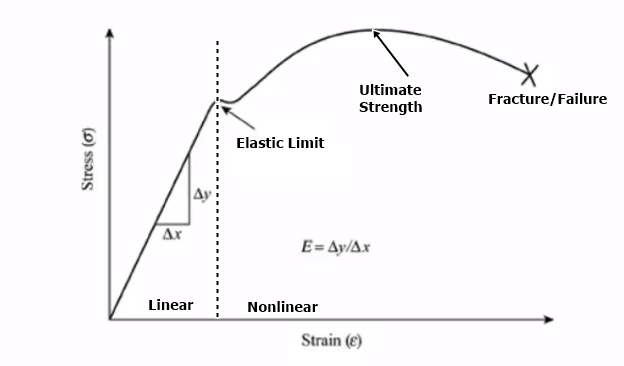Figure 1: stress-strain curve for the ductile materials

Material nonlinearity involves the nonlinear behavior of a material based on a current deformation, deformation history, rate of deformation, temperature, pressure, and so on. Examples of nonlinear material models are large strain (Visco) elasto-plasticity and hyperelasticity (rubber and plastic materials) and other types.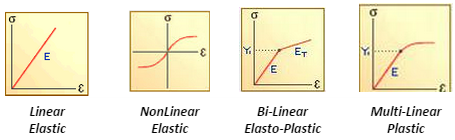Figure 2:  Different ways of representing the material properties

In this, elasto-plastic material is the simplest of all nonlinear material models as shown above fig 2. It considers that once the yield stress is reached, stress becomes constant and strain continues to increase. These models (von-mises or Tresca) work well for material for which a strain-stress curve shows a ‘plateau’ before reaching the ultimate stress. Most engineering metals and some plastics are well-characterized by this material model. This model works for soils and granular materials (Drucker-Prager)

Stress-strain for structural steel will consist of elastic and perfectly plastic or strain hardening region. We call this kind of material elastoplastic material. Analysis of structures on the basis of the elasto-plastic diagram is called elastoplastic analysis. Bilinear stress-strain diagram having different slopes is sometimes used to approximate the general nonlinear diagrams in figure 3. This will include strain hardening.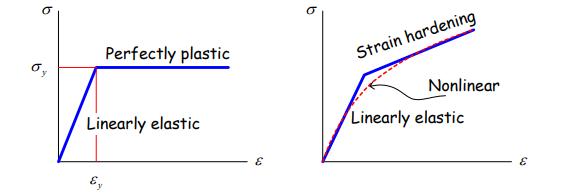Figure 3:  Perfectly plastic and Strain hardening (Non-Linear)

Brittle material: Materials that do not exhibit yielding before failure.

Some materials will show both ductile and brittle behaviour, e.g., steel with high carbon content will demonstrate brittle behaviours while steel with low carbon content will be ductile or steel subjects to low temperature will be brittle while those in the high-temperature environment will be ductile.

To define the slope of the plastic region where stress is not proportional to strain i.e., elastic-plastic region is represented in terms of tangent modulus. The tangent modulus is always less than the young’s modulus. The tangent modulus is defined as the slope of a line tangent to the stress-strain curve at a point of interest. Tangent modulus can have different values depending on the point at which it is determined. For example, outside the linear elastic region, at point A shown, for example, the tangent modulus is always less than Young's modulus. The tangent modulus is mostly used to describe the stiffness of a material in the plastic range, and it is denoted by Et.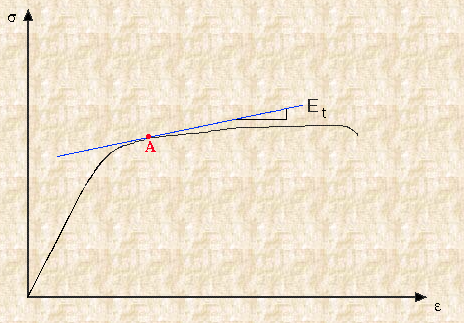The tangent modulus is useful in describing the behavior of materials that have been stressed beyond the elastic region. When a material is plastically deformed there is no longer a linear relationship between stress and strain. The tangent modulus quantifies the "softening" or "hardening" of material that generally occurs when it begins to yield.

Although the material softens it is still generally able to sustain more load before ultimate failure. Therefore, a more weight-efficient structure can be designed when plastic behavior is considered. For example, a structural analyst may use the tangent modulus for the simulation of nonlinear material analysis.

The tangent-modulus theory oversimplifies the inelastic by using only one tangent modulus. In reality, the tangent modulus depends on the stress, which is a function of the bending moment that varies with the displacement w.

Calculation of tangent modulus:

In general, for any material, no data will be available for the tangent modulus. For almost all the materials, the three parameters normally available are YIELD STRESS (YS) , ULTIMATE STRENGTH (UT) and STRAIN AT RUPTURE (ER) or Elongation at point 2. Using this information, we have to estimate the tangent modulus TA as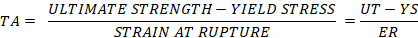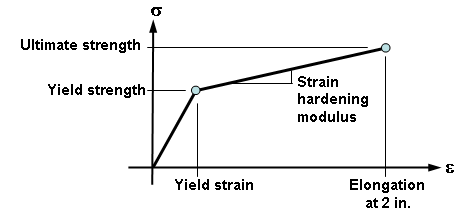Figure 4: Formulation and graph for the tangent modulus calculation

Multilinear properties:

This is the more advanced option than the bilinear material described previously. A lot of settings remain the same (yield criterion, hardening rule, initial yield point) except you need to provide different modules according to the slope considered per the stress-strain curved. That means, we are making the curved stress-strain curve as shown in the fig is divided into pieces and made as to the linear line. These linear portions will have different modulus according to the slope of the line where stress is not proportional to strain.

Till yield i.e., the elastic region is the same in all the cases – linear, bi-linear, multilinear. After the yield point in the nonlinear material analysis, according to the data available one should go for bilinear or multilinear materials properties.

For example, the curve for multi-linear is as shown in the figure and the stress-strain curve for this type of material must be defined starting in (ε=0; σ=0) point. The second point on the curve should be at initial yield (ε1; σy) for von Mises and Tresca. The process repeats in the same way according to the data availability. Always we get the data for multi-linear material properties from the experiments conducted on the specific material specimen.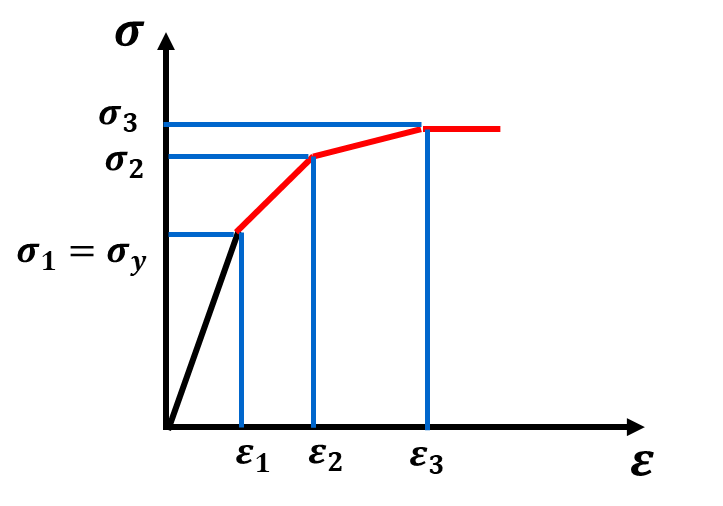Figure 5: Multi-linear stress-strain curve with various slopes

To conclude in a simple statement, in most of the cases for the material non-linear, we generally use bi-linear material properties and multi-linear is used where we have concise material data.

Rules for input MP’s:

• It is not possible to input both limits of proportionality and the elastic limit. The user has to make a choice.

• As a rule, the first data point is taken as the yield stress.

• The first slope has to match the modulus of elasticity.

• Both the stress and strain data should be monotonically increasing.

• To give bilinear material properties, one needs to enter elastic properties prior

• Incremental slopes should be steadily increasing and should follow a downward slope after reaching ultimate stress.

• Plastic material properties are less than the elastic properties both in tangential &Multi linear cases (stress-strain curve)

• Brittle material has ultimate and fractures at same location i.e., it doesn’t have plastic zone

Related Blog1 year ago / 0

Supports are arguably one of the most important aspects of a structure, as it specifies how the forc…1 year ago / 0

Petrol Vs Diesel Engine Engines are the powerhouse of vehicles and systems. It provides the energ…